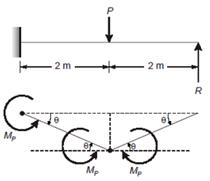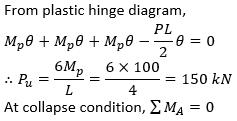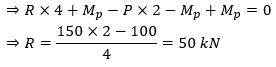# Civil Engineering (CE) : Mock Test For GATE

## 65 Questions MCQ Test Mock Test Series for Civil Engineering (CE) GATE | Civil Engineering (CE) : Mock Test For GATE

Description
This mock test of Civil Engineering (CE) : Mock Test For GATE for GATE helps you for every GATE entrance exam. This contains 65 Multiple Choice Questions for GATE Civil Engineering (CE) : Mock Test For GATE (mcq) to study with solutions a complete question bank. The solved questions answers in this Civil Engineering (CE) : Mock Test For GATE quiz give you a good mix of easy questions and tough questions. GATE students definitely take this Civil Engineering (CE) : Mock Test For GATE exercise for a better result in the exam. You can find other Civil Engineering (CE) : Mock Test For GATE extra questions, long questions & short questions for GATE on EduRev as well by searching above.
QUESTION: 1

###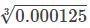is equal to

Solution: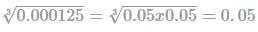QUESTION: 2

### Natural numbers from 1 to 999 are written in a random manner on a board. Each time 2 numbers are chosen and their product is written on the board after erasing the 2 numbers. After 999 such operations, which of the following is likely to be true?

Solution:

There are total 1000 numbers. Each time 2 numbers are erased and replaced with their product, one number is getting reduced. After 999 such operations, 999 numbers would have got erased leaving behind exactly 1 number which means option ‘a’ or ‘b’ or ‘c’.

QUESTION: 3

### Assume that equal number of people are born each day of the year. If the century under consideration is the 20th century, what is the percentage of people who are born on 29th February? [Assume no deaths occur during the period under consideration].

Solution:

In 20th century i.e. 1901 to 2000, there are 25 leap years.
The total number of people born on 29th February = 25p and the total number of people born in entire 20th century = 36500p + 25p = 36525p; giving the required percentage of people born on 29th February = (25p/36525p) x 100 = 0.0684
Caution: A common error likely to be committed is to divide 25p by 365p which will give the required percentage as 0.0685 which is also one of the choices OR forget to multiply 25p/36525p by 100 getting a value = 0.000684 which also happens to be one of the options.

QUESTION: 4

Four distinct amounts i.e. Rs. 3000, Rs. 4000, Rs. 6000 and Rs. 7000 are put on deposit on simple interest @ 4%, 6%, 7.5% and 8% per annum - each amount having been put on deposit at a different rate of interest. It is also known that the highest principal amount has not been put on deposit at the lowest rate of interest and vice versa; likewise It is also known that the highest principal amount has not been put on deposit at the highest rate of interest and the lowest principal amount has not been put on deposit at the lowest rate of interest. If the highest principal amount does not earn maximum interest, what is the rate of interest (in %) for Rs.7000

Solution: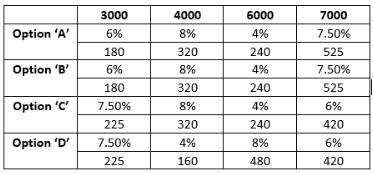Based on the table which lists the possible options, we can clearly eliminate options ‘A’, ‘B’ and ‘C’. Rs. 7000 earns maximum interest which violates the given condition.
This leaves option ‘D’ and in this case, Rs. 7000 is not earning highest interest which leads us to the required answer i.e. option ‘d’.

QUESTION: 5

P and Q are distinct real numbers such that 4 < P < 5 and -9 < Q < -8. Which of the following has the least possible value?

Solution:

In order to get the least value, we must choose the most negative value with ODD index AND most positive value for P. This rules out option ‘d’. Now if simply put the most positive and most negative values for P and Q, we end up getting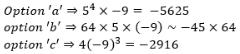Option d will be a positive number.
So option a will be the smallest number

QUESTION: 6

The greatest among the numbers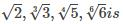Solution: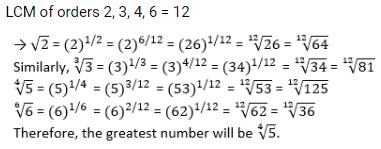QUESTION: 7

At what time between 6 a.m. and 7 a.m will the minute hand and hour hand of a clock make an angle closest to 60°?

Solution: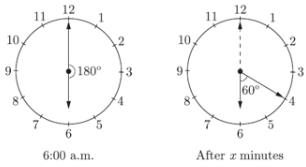As shown in Figure above, at 6:00 a.m. initial angle between minute and hour hand is 180o.As we know that hour hand completes 30° in every hour (60 minutes), so angle moved by hour hand in 1 minute is 30/60. Similarly, minute hand complete 360° in every hour, so angle moved by minute hand in 1 minute is 360/60. Let us assume that after x minutes the angle is 60°. Let us assume that after x minutes the angle is 60°.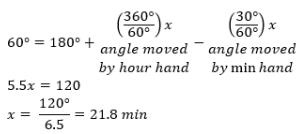QUESTION: 8

Raja and joseph start from their hostel. The distance covered by Raja in 3 steps is identical to the distance covered by joseph in 4 steps while the time taken by both of them to cover a step is identical. At a particular instance of time when both of them had covered 800 steps each, the distance between them was 300 m. Now, raja cuts down the length of his step by 1/3rd and Joseph increase the length of his step by 20% with time taken to cover a step by both remaining identical. Both will be at the same spot at the same time in _________ more steps.

Solution:

Let R and J be the length of each step of Raja and Joseph respectively.
We can write the following equations based on the given information:
3R = 4J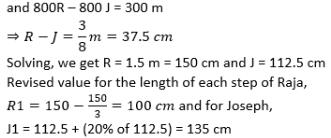Now, the difference in the length of a step of Raja and Joseph = 35 cm with Joseph's step being longer. In order to catch up with Raja who is ahead of him by 300 m, the number of steps required
= 300/0.35 = 857.14 ≌ 857

*Answer can only contain numeric values
QUESTION: 9

If f(1) = 1, f’(1) = 2, then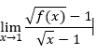is

Solution:

Applying L’ Hospital’s rule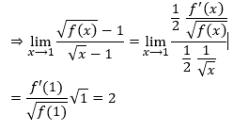QUESTION: 10

In the following question, a sentence is given with a blank to be filled in with appropriate word(s). Four alternatives are suggested for the blank. Choose the correct alternative out of the four.  Wider international attention can be expected _______ continue, potentially exacerbating political polarization at every step.

Solution:

The preposition ‘to’ indicates concern or likely to concern something or approaching or reaching (a particular condition). In this case, it is the expected attention.
by identifies the agent performing an action.
for as a preposition has multiple contextual
sages, none of which fits here.
too means to a higher degree than is desirable, permissible, or possible; excessively.
Hence, the correct answer is option b.

QUESTION: 11

Direction: In the following question consists of two statements Assertion(A) and Reason(R). You have to read the statements carefully and select the answers to these questions using the options below.
Assertion (A): a material is incompressible if its poissions ratio is 0.5
Reason(R): the bulk modulus K is related to modulus of elasticity E and to poissons ratio as per well known relationship.

Solution:

Most materials have poissons ratio values ranging between 0 and 0.5. a perfectly incompressible material deformed elastically at small strains would have poissons ration of exactly 0.5 at this value material is incompressible.

QUESTION: 12

The ground water is pumped through an aquifer having an area of 150 Hectares which has a porosity of 0.4. Specific retention of the medium is 0.25. Find the change in the ground water storage when the water table drops from 15 m to 25 m.

Solution:

Given,
η = 0.4
γR = 0.25
γS = 0.4 – 0.25 = 0.15
γS = Amount of water drained/Amount of medium
Amount of water drained = 0.15 × 150 × 10 = 225 Ha-m

QUESTION: 13

Let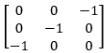The only correct statement about the matrix A is

Solution: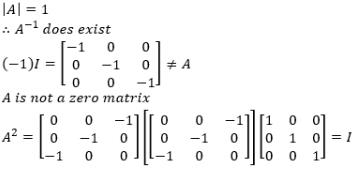QUESTION: 14

The ultimate load capacity of a 10 m long concrete pile of square cross section 500 mm × 500 mm driven into a homogenous clay layer having undrained cohesion value of 40 kPa is 700 kN. If the cross section of the pile is reduced to 250 mm × 250 mm and the length of the pile is increased to 20m, the ultimate load capacity will be

Solution: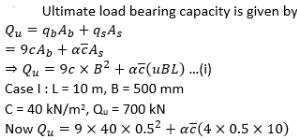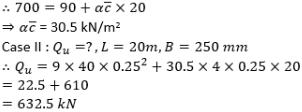QUESTION: 15

The result of Vee-Bee test is expressed in terms of:

Solution:

The main objective of Vee-Bee test is to determine the workability of the freshly mixed concrete.
Vee-bee test carries out the relative effort measurement to change the mass of the concrete from a definite shape to the other. That is, as per the test, from the conical shape to the cylindrical shape by undergoing vibration process. The time required for the complete remolding is a measure of the workability and is expressed in the Vee-Bee seconds.

QUESTION: 16

A catchment having an area of 150 km2 has the ø index 0.3 cm/hr. The base flow at the outlet is 10m3/s. One hour unit hydrograph of the catchment area is triangular in shape with a time base of 20 hours. The peak ordinate occurs at 8 hours.
The peak ordinate of the unit hydrograph (m3/s/cm) is.

Solution:

Total depth of rainfall = 1 cm = 0.01 m
Total runoff volume = Area of hydrograph = 1/2 x time base x peak ordinate
0.01 x 150 x 106 = 0.5 x ( 20 x 3600) x peak ordinate
Peak ordinate = 41.66

QUESTION: 17

The value of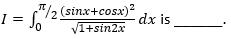Solution: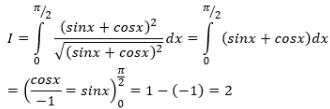QUESTION: 18

A particular section of steel shaft is subjected to maximum bending stress of 160 MPa and a maximum shear stress equal to 60 MPa. The yield point in tension of the material is 400 MPa. If maximum shear stress theory is used, the FOS will be _____________.

Solution: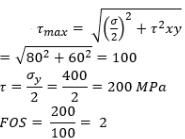QUESTION: 19

Match List-I (Item) with List-II (Features) and select the correct codes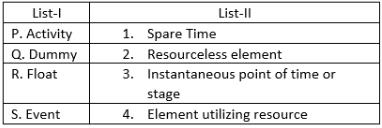Solution:

• In project management, a task is an activity that needs to be accomplished within a defined period of time or by a deadline to work towards work-related goals.
• A dummy activity is a simulated activity of sorts, one that is of a zero duration and is created for the sole purpose of demonstrating a specific relationship and path of action on the arrow diagramming method.
• Float, sometimes called slack, is the amount of time an activity, network path, or project can be delayed from the early start without changing the completion date of the project.
• An event is an occurrence or an outcome which is of significance and based on which typically, a business rule can be triggered. For an example, an event could be the start of an activity, end of an activity, non-completion of an activity within a certain timeframe, etc.
So, correct matching are as follows
• Activity - Element Utilizing Resource
• Dummy - Resourceless element
• Float - Spare Time
• Event - Instantaneous point of time
d option is correct!

QUESTION: 20

Consider a small length of a beam subjected to simple bending. It has two sections AB and CD normal to the axis of the beam N-N. The amount by which the layer increases or decreases in length,

Solution: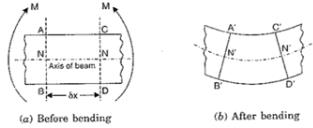The axis of the beam is the neutral axis.
The amount by which a layer increases or decreases in length, depends upon the position of the layer w.r.t. N-N.
In the above fig. as we move from top layer to N-N, the length of the layer increases. While moving from bottom layer to N-N, the length of the layer decreases.

QUESTION: 21

In a two-dimensional stress analysis, the state of stress at a point is shown below. If σ = 120 MPa and τ = 70 MPa, then σx and σy are respectively. (AB=4, BC=3, AC=5)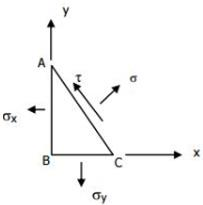Solution: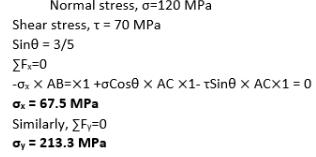QUESTION: 22

A steel rod of circular cross-section is to carry a tensile load P = 150 kN. The allowable working stress in shear for the rod is τallowable = 55 MPA. The required diameter for the rod is

Solution: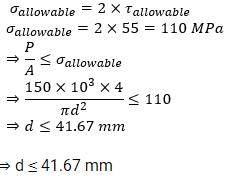QUESTION: 23

Match List I and List II and select the correct answer using the codes given below the lists.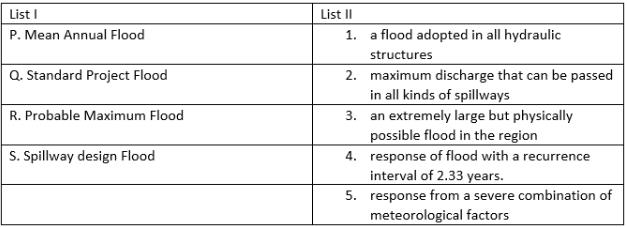Solution:

Probable maximum flood is the extreme flood that is practically possible in a region as a result of severe most combination.
The standard Project flood results from severe combinations of meteorological and hydrological factors that are reasonably applicable in that region.
According to Gumbel’s distribution, the value of flood with return period of 2.33 years is the mean annual flood.

QUESTION: 24

The displacement of joint 4 relative to joint 1 along the direction 1-4 of the truss given below. Axial rigidity of all member is AE.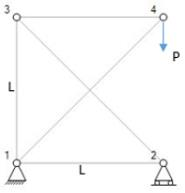Solution:

Calculation of K force due to application of unit force in the direction of 1-4.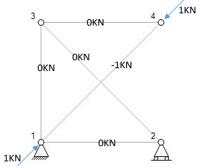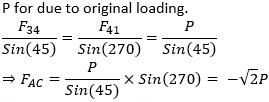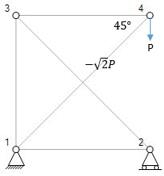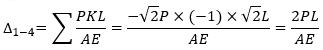QUESTION: 25

The maximum tensile stress at the section X–X shown in the figure below is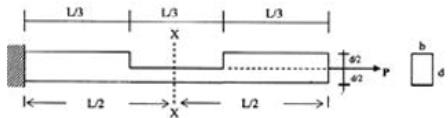Solution:

This is a case of combined direct and bending stress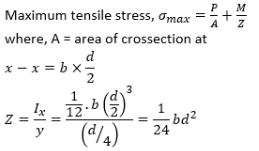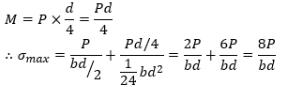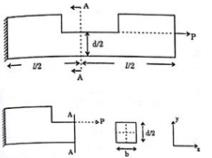QUESTION: 26

The variation of rigidity factor for a tyre pressure is stated as:

Solution:

The variation of rigidity factor for a tyre pressure is stated as:
<1 for tyre pressure greater than 7 kg/cm2
>1 for tyre pressure less than 7 kg/cm2
=1 for tyre pressure equal to 7 kg/cm2

QUESTION: 27

Using IRC: 37 - 1984 “Guidelines for the Design of Flexible Pavements” and the following data, choose the total thickness of the pavement.
No. of commercial vehicles when construction is completed= 2723 veh/day
Annual growth rate of the traffic = 5.0%
Design life of the pavement = 10 years
Vehicle damage factor = 2.4
CBE value of the subgrade soil = 5%
Data for 5% CBR value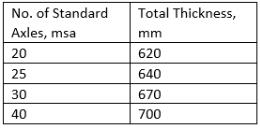Solution: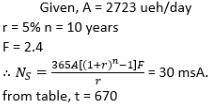QUESTION: 28

Each of the following items consists of two statements, one labelled as the ‘Statement (I)’ and the other as ‘Statement (II)’. Examine these two statements carefully and select the answers to these items using the codes below:
Statement (I): A moving hydraulic jump is called a surge.
Statement (II): The travel of a wave is faster in the upper portion than in the lower portion in case of positive surges.

Solution:

A surge in an open channel is a sudden change of flow depth (i.e. abrupt increase or decrease in depth). An abrupt increase in flow depth is called a positive surge while a sudden decrease in depth is termed a negative surge.
So, Statement II is not the correct explanation of the statement I

QUESTION: 29

A rigid body shown in the Fig(a) has a mass of 10 kg. It rotates with a uniform angular velocity 'ω' A balancing mass of 20 kg is attached as shown in Fig(b) . The percentage increase in mass moment of inertia as a result of this addition is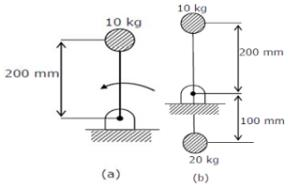Solution:

Given: First Mass, mi = 10 kg
Balancing Mass, m2 = 20 kg
We know the mass moment of inertia, I = mk2
Where, k = Radius of gyration
Case (I): When mass of 10 kg is rotates with uniform angular velocity 'ω'
The moment of inertia I1= m1k12
I1 = 10 x (0.2)2 k1= 200 mm = 0.2 meter
= 10 x 0.04 = 0.4 kg m2
Case (II): When balancing mass of 20 kg is attached then moment of inertia
I2 = 10 x (0.2)2 + 20 x (0.1)2 Here ;Q. = 0.2m
= 0.4 + 0.2 = 0.6 and k2= 0.1m
Percent increase in mass moment of inertia,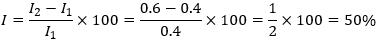*Answer can only contain numeric values
QUESTION: 30

The characteristic compressive strength of concrete required in a project is 25 MPa and standard deviation in the observed compressive strength expected at site is 4 MPa. The average compressive strength of cubes tested at different water-cement (w/c) ratios using the same material as is used for the project is given in the table.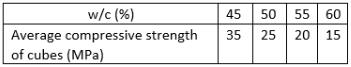The water-cement ratio (in percent, round off to the lower integer) to be used in the mix is________

Solution:

Here,
fck = 25 ; σ = 4 MPa
and
fm =fck + 1.65σ = 31.6 MPa
Using interpolation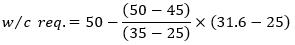= 46.7

QUESTION: 31

The initial setting time of cement depends most on

Solution:

The compound C3A is characteristically fast reacting with water and may lead to an immediate stiffening of paste, and this process is termed flash set. Hence, initial setting time of cement depends most on C3A.

QUESTION: 32

For a given matrix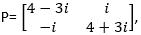Where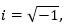the inverse of matrix p is

Solution: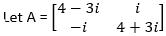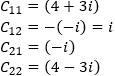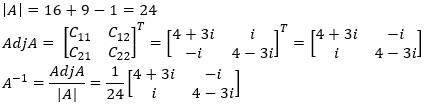QUESTION: 33

Two rigid bodies of mass 5 kg and 4 kg are at rest on a frictionless surface until acted upon by a force of 36 N as shown in the figure. The contact force generated between the two bodies is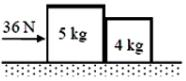Solution: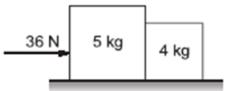As after action of 36 N, both blocks will move with same acceleration so considering 5 kg and 4 kg together in a system and applying Newton’s 2nd law.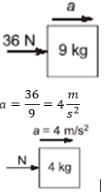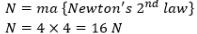QUESTION: 34

What is the ultimate capacity in kN of the pile group shown in the figure assuming the group to fail as a single block?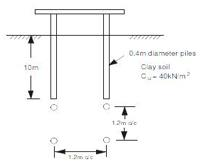Solution:

Given,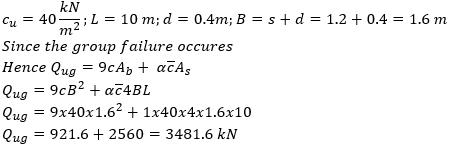QUESTION: 35

The speed and delay studies on a defined section of highway are conducted by

Solution:

Speed and delay studies are useful in detecting the spots of congestion.
There are various methods of carrying out speed and delay study.
(i) Moving car or floating car method.
(iii) Interview method
(iv) Elevated observation
(v) Photographic technique

QUESTION: 36

The figure below shows a fixed beam of elastic material possessing sufficient ductility and of a uniform cross-section. It carries a uniformly distributed load which is gradually increased to its ultimate value Wu per unit length, at which the beam is transformed into a failure mechanism. What is the magnitude of Wu in terms of the ultimate moment Mu of the beam-section and span L?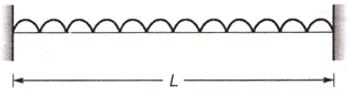Solution: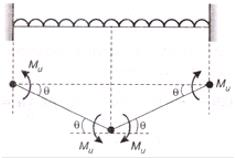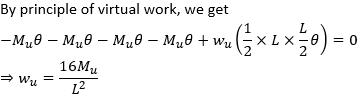QUESTION: 37

A pile of 0.50 m diameter and of length 10 m is embedded in a deposit of clay. The undrained strength parameters of the clay are cohension= 60 kN/m2 and the angle of internal friction = 0. The skin friction capacity (kN) of the pile for an adhesion factor of 0.6, is

Solution:

Skin friction capacity of clay is given by
Qs = αCAs
where, As = effective area in developing friction
= π × d × 1

*Answer can only contain numeric values
QUESTION: 38

The product of Eigen values of the matrix P is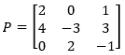Solution: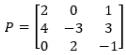We know that, product of eigen values of P = determinant of P = 2(3-6) - 0 + 1 (8) = -6 + 8 = 2

QUESTION: 39

The high alumina cement has certain properties as mentioned below:
1) High alumina cement has high early compressive strength and high heat of hydration than ordinary Portland cement
2) High alumina cement is not suitable to be used in cold regions.

Which of the following statement (s) correct?

Solution:

The high alumina cement attains a strength of 30 N/mm2 after one day and emits high heat of hydration
Due to high early compressive strength, it is suitable to be used in cold regions.

QUESTION: 40

The eigen values of the following matrix are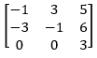Solution:

Eigen values are given by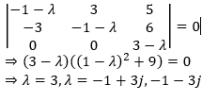QUESTION: 41

Consider the following PERT network: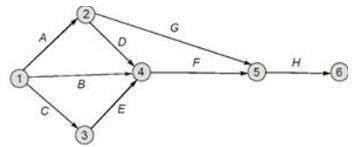The optimistic time, pessimistic time and most likely time of all the activities are given in the table below: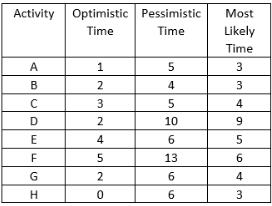The critical path duration of the network (in days) is

Solution:

The network of the project is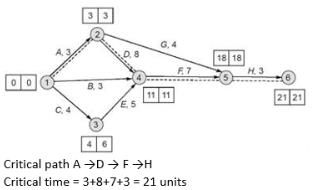QUESTION: 42

Statement 1: to test a brittle material, maximum principle stress theory is suitable.
Statement 2: Maximum Principle stress theory is not good for mild steel body.

Solution:

Since brittle materials are weak in tension, maximum principle stress theory is suitable for brittle components
Ductile materials may undergo shear failure, thus making this theory not suitable for ductile components.

QUESTION: 43

Which of the following is added for quick setting of cement?

Solution:

The quick setting cement is manufactured by adding a small percentage of aluminium sulphate and then it is finely grinded with cement. And also for quick setting action a small percentage of gypsum or retarder is added. The aluminium sulphate is mainly added to accelerate the setting time fast.
Gypsum or plaster of paris plays a very important role in controlling the rate of hardening of the cement. During the cement manufacturing process, upon the cooling of clinker, a small amount of gypsum is introduced during the final grinding process. Gypsum is added to control the “setting of cement”.

QUESTION: 44

To obtain the lake evaporation in cm, the evaporation observed from an ISI pan under similar climatic and exposure condition is found to be 12.60 cm. The lake evaporation will be in the range of

Solution:

We know that Lake evaporation = Cp x Pan Evaporation
In case of ISI pan, CP = 0.80
So lake evaporation = 0.80 x 12.60 x 10 = 100.8 mm

QUESTION: 45

Following data are given:
1. Available moisture holding capacity of soil=12.5cm/m depth of soil
2. Root zone depth of crop= 0.8m
3. Consumptive use= 5mm/day
If moisture depletion is allowed to 50% of available moisture then the frequency of irrigation will be ___days.

Solution:

Total available moisture holding capacity of soil=12.5X0.8=10 cm=100 mm.
Moisture which must be added to the soil through irrigation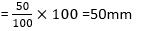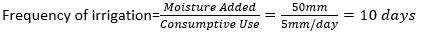QUESTION: 46

Which of the following represents steady flow

Solution:

As in case of steady flow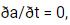where a represents the flow characteristics like velocity, depth, etc. And it represents the time. In case of steady flow these flow characteristics may vary with distance along the line of flow.

QUESTION: 47

A steel portal frame has dimensions, plastic moment capacities and applied loads as shown in the figure. The vertical load is always 2.5 times of the horizontal load. The collapse load W of a beam mechanism is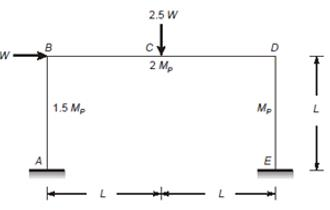Solution:

The ends B and D of the frame ABDE are fixed joints. Therefore, the beam BD acts as a fixed end beam.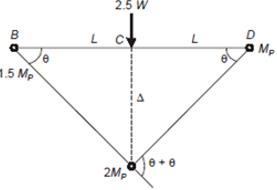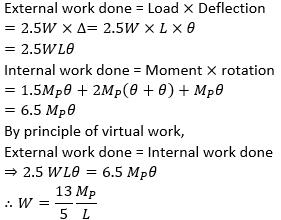QUESTION: 48

A square pile group of 9 piles having depth of 9 m passes through a recently filled up material of 4.5 m depth. The diameter of the pile is 30 cm and pile spacing is 60 cm centre to centre. If the unconfined compressive strength of the cohesive material is 60 kN/m2 and unit weight is 15 kN/m3, the negative skin friction of the pile group is ________ kN.

Solution:

D' = Depth of soil fill = 4.5 m Individual piles: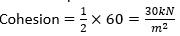Negative skin friction, Qng = n x PD'c
= 9 x π x 0.3 x 4.5 x 30 =1145.11 kN
Pile group: B = 2S + d
=2x0.6+0.3=1.5m
Perimeter = 4B = 6m
Area = B2 = 2.25 m2
Negative skin friction,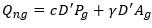= 30 X 4.5 X 6 + 15 X 4.5 X 2.25
= 810 + 151.875
= 961.875 kN .
Negative skin friction (the larger of the two values) = 1145.11 kN

QUESTION: 49

Find the force in the member BF if three horizontal forces of 1 kN, 2 kN and 3 kN are applied respectively on B, C and D.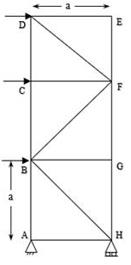Solution:

Cut a section horizontally.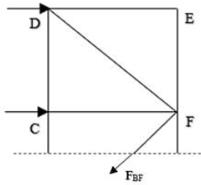From equillibrium of horizontal forces,
Fc+ Fo — FBF sin 45 = 0
3+2 = FBF sin 45
FBF = 5√2
It is +ve means tensile

*Answer can only contain numeric values
QUESTION: 50

The flood discharge with a return period of 500 yr at a particular community was 20000 m3/s with a probable error of 1850 m3/s. The 90% confidence probability limits of the 500 yr flood by Gumbel's method with f (c) as 1.645 is______ m3/s. (Roundoff to nearest integer)

Solution:

For a confidence probability c, the confidence interval of the XT bounded by intervals X1 and X2 is given by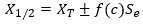f(c) = function of the confidence probability;
Se = probable error
X1 = 20000 — 1.645(1850)
X1 = 16956.75 m3/s
X2 = 20000 + 1.645(1850) = 23043.25 m3/s

QUESTION: 51

What would be the equivalent stress (MPa) in simple tension according to maximum principal strain theory. If At a point in a structure there are two mutually perpendicular tensile stresses of 600 MPa and 200MPa at failure. poisson’s ratio is 0.25.

Solution:

According to max. principal strain theory,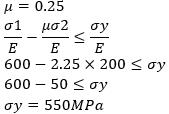QUESTION: 52

The two main compounds imparting strength for Ordinary Portland Cement are:

Solution:

C3S and C2S together constitute about 70% of cement and are responsible for providing most of the strength to it C3A is responsible for flash set and C4AF generates very less strength.

QUESTION: 53

Consider the following types of cements:
1) Portland pulverized fuel ash cement
2) High alumina cement
3) Ordinary Portland cement
4) Rapid hardening cement

Which one of the following is the correct sequence of the above types of cement in terms of their increasing rate of strength gain?

Solution:

The increase in strength of cement with time depends on the C2S content of cement.
• Portland pulverized fuel ash cement has a lower rate of strength gain as compared to OPC.
• The 1-day strength of RHC is about 3-day strength of OPC with the same w/c ratio.
High Alumina cement achieves about 20% of the ultimate strength within 24hrs.

QUESTION: 54

The precedence relations and duration (in days) of activities of a project network are given in the table. The total float (in days) of activities e and f, respectively, are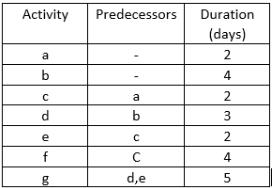Solution: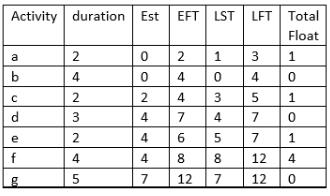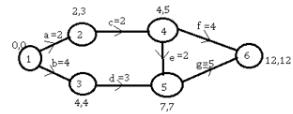Total Float = LFT – EFT =LST- EST
Where,
EST is the Earliest Short Time
EFT is Earliest Finish time
LST is Latest start time
And, LFT is Latest Finish time

QUESTION: 55

A Francis turbine working at 400 rpm has a unit speed of 50 rpm and develops 500 kW of power.What is the effective head under which this turbine operates?

Solution:

Unit speed of the turbine is given by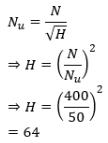QUESTION: 56

Tricalcium aluminate (C3A)

Solution:

Descending order of heat of hydration C3A>C3S > C4AF >C2S, C3A undergoes hydration
Within 24 hours after addition of water in
Cement. It is responsible for initial setting
But not for early strength.

QUESTION: 57

Given two complex numbers,  z1 = 5+5 √3iandz2 = 2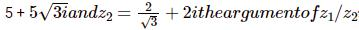in  degrees is

Solution: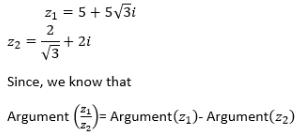QUESTION: 58

Two major roads with two lanes each are crossing in an urban area to form an un–controlled intersection. The number of conflict points when both road are one–way is “X” and when both roads are two–way is “Y”. The ratio of X to Y is

Solution:

Number of conflict points for one-way roads = 6(x)
Number of conflict points for two-way roads= 24(y)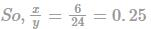QUESTION: 59

The volume of an object expressed in spherical co-ordinates is given by;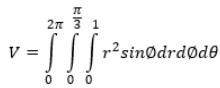The value of the integral is

Solution: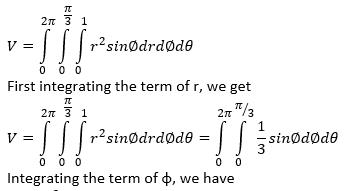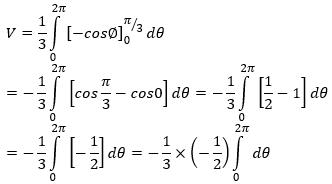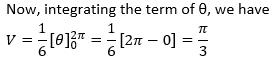QUESTION: 60

The diaphragm of a tachometer are 3 in number spaced at 1.25mm. The distance from the object glass and the trunnion axis is 0.11m with the focal length of the object glass as 240 mm. Considering that a convex lens is used in the externally focusing telescope, compute the constants(multiplying & additive) using the principle of optics.

Solution:

Given f = 24cm; d = 11cm
i = 1.25mm = 0.125cm
Based on the principle of optics, the constants are
(f/i) and (f +d)
A convex lens used in the external focusing telescope is the analytic lens, which indicates that the additive constant is Zero.
Multiplying constant = f/i = 24/0.125 = 192
Additive constant = (f+d) = 0

QUESTION: 61

The state of 2D–stress at a point is given by the following matrix of stress: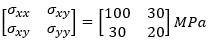What is the magnitude of maximum shear stress(Radius of Mohr's circle) in MPa?

Solution: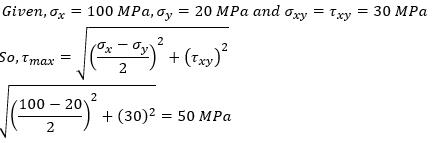QUESTION: 62

That extreme flood that is physically possible in a region from a severe-most combination, including rare combination of metrological and hydrological factors, is designated as the

Solution:

Maximum Probability Flood (MPF) differs from the Standard Project Flood (SPF) in that it includes the extremely rare and catastrophic floods and is usually confined to spillway design of very high dams. The SPF is generally around 80% of the MPF for the basin.

QUESTION: 63

Most economical circular section is designed for

Solution:

Most economical section gives maximum discharge for a given amount of excavation and the discharge is maximum velocity is maximum, the area of cross section remaining constant. And for a given slope and roughness coefficient the velocity is maximum when the hydraulic radius (area / perimeter) must be maximum. For it to be maximum the perimeter should be minimum.
But for conduits the condition of the area remaining constant does not hold good since both the wetted perimeter and the wetted area changes with depth of flow, so for most economical section in case of open channel flow in circular conduits is designed for
A) Maximum mean velocity
B) Maximum flow rate

QUESTION: 64

The 2 kg mass C moving horizontally to the right, with a velocity of 5 m/s, strikes the 8 kg mass B at the lower end of the rigid massless rod AB. The rod is suspended from a frictionless hinge at A and is initially at rest. If the coefficient of restitution between mass C and mass B is one, determine the angular velocity of the rod AB immediately after impact.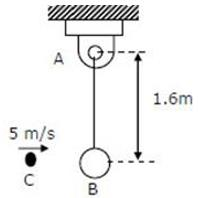Solution: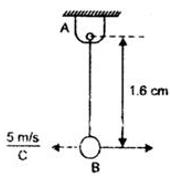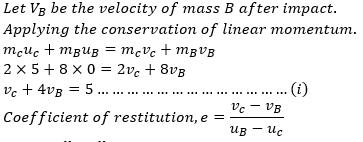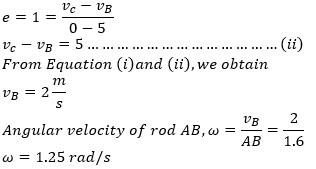QUESTION: 65

The magnitude of load P is increased till collapse and the plastic moment carrying capacity of steel beam section is 100 kN/m. The value of R (in kN) using plastic analysis is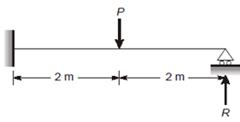Solution: